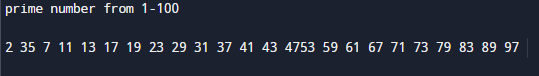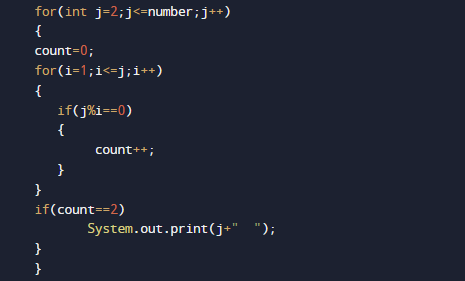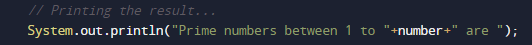# Java Program to display first 100 prime numbers

In this tutorial you will learn about the Java Program to display first 100 prime numbers and its application with practical example.

In this tutorial, we will learn to create a Java Program to display first 100 prime numbers using Java programming.

## Prerequisites

Before starting with this tutorial we assume that you are best aware of the following Java programming topics:

• Java Operators.
• Basic Input and Output function in Java.
• Class and Object in Java.
• Basic Java programming.
• If-else statements in Java.
• For loop in Java.
• User Define functions

## What is Prime Number?

A  number is called a Prime number if a number is divisible by 1 or itself only.
For example 2, 3, 5, 7, 11, 13, 17..are some prime numbers.

## Java Program to display prime numbers between 1 and 100 or 1 and n

In this program we would  print the prime numbers between 1 and 100 .First of all we declare variables and then find prime number from 1 to 100.Let have a look at the code.

## Output

Prime number From 1 – 100.In the above program, we have first declared and initialized a set variables required in the program.

• count=  it will show number is prime or not
• i and j = for iteration.

After declaring variables we take a value from user and print prime number from 1 – N.

### Algorithm

• 1: START
• 2: declare variables count =0, i=1,j=1.
• 3: SET j= 1
• 4: SET count = 0
• 5: if i%j = = 0 then count ++
• 6: j = j + 1
• 7: if count= 2 then print the value of i.
• 8: i = i +1
• 9: ENDSimply here we check each and every value form 1 to 100 and print only values those satisfied the logic of a prime number it means number which divisible by itself only are prime numbers..and in that manner we print all prime number lies between 1 -100 in  our program.

In this tutorial we have learn about the Java Program to display first 100 prime numbers and its application with practical example. I hope you will like this tutorial.